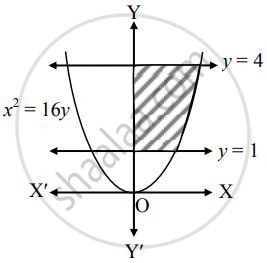# Area of the region bounded by x2 = 16y, y = 1 and y = 4 and the Y-axis, lying in the first quadrant is _______. - Mathematics and Statistics

MCQ
Fill in the Blanks

Area of the region bounded by x2 = 16y, y = 1 and y = 4 and the Y-axis, lying in the first quadrant is _______.

#### Options

• 63 sq. units

• (3)/(56)"sq. units"

• (56)/(3)"sq. units"

• (63)/(7)"sq. units"

#### Solution

Area of the region bounded by x2 = 16y, y = 1 and y = 4 and the Y-axis, lying in the first quadrant is bbunderline((56)/(3)"sq. units").

Explanation:Required area = int_1^4x*dy

= int_1^4 4sqrt(y)*dy

= 4[y^(3/2)/(3/2)]_1^4

= (8)/(3)[(4)^(3/2) - (1)^(3/2)]

= (8)/(3)(8 - 1)

= (56)/(3)"sq.units".

Is there an error in this question or solution?
Chapter 7: Applications of Definite Integration - Miscellaneous Exercise 7 [Page 157]

#### APPEARS IN

Balbharati Mathematics and Statistics 1 (Commerce) 12th Standard HSC Maharashtra State Board
Chapter 7 Applications of Definite Integration
Miscellaneous Exercise 7 | Q 1.3 | Page 157
Share